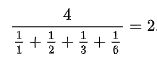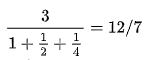# Check if the given number is Ore number or not

Given a positive integer n, check if it is an Ore number or not. Print ‘YES’ if n is an ore number otherwise print ‘NO’.

Ore Number : In mathematics, Ore numbers are positive integers whose divisors have a integer harmonic value. Ore numbers are often called as harmonic divisor number. Ore numbers are named after Øystein Ore.

For example, 6 has four divisors namely 1, 2, 3 and 6.
Harmonic mean of the divisors is-The harmonic mean of divisors of 6 is 2, an integer. So, 6 is an Ore number or harmonic divisor number.

First few Ore numbers or harmonic divisor numbers are:

1, 6, 28, 140, 270, 496, 672, 1638, 2970, 6200, 8128, 8190

Examples:

```Input : N = 6
Output : Yes

Input : N = 4
Output: No
Explanation : Harmonic mean of divisors of 4
is not an Integer.```

## Recommended: Please try your approach on {IDE} first, before moving on to the solution.

Prerequisite:

The idea is to generate all divisors of the given number and then check if the harmonic mean of the divisor is an Integer or not.

1. Generate All Divisors of the given number – ‘n’
2. Calculate Harmonic mean of the divisors of n
3. Check if Harmonic mean is an Integer or not
4. If Yes, Then the number is an Ore Number otherwise Not

Below is the implementation of above approach:

## C++

 `// CPP program to check if the given number is ` `// Ore number ` ` `  `#include ` `using` `namespace` `std; ` ` `  `vector<``int``> arr; ` ` `  `// Function that returns harmonic mean ` `void` `generateDivisors(``int` `n) ` `{ ` `    ``// Note that this loop runs till square root ` `    ``for` `(``int` `i = 1; i <= ``sqrt``(n); i++) { ` `        ``if` `(n % i == 0) { ` ` `  `            ``// If divisors are equal, store 'i' ` `            ``if` `(n / i == i) ` `                ``arr.push_back(i); ` ` `  `            ``else` `// Otherwise store 'i' and 'n/i' both ` `            ``{ ` `                ``arr.push_back(i); ` `                ``arr.push_back(n / i); ` `            ``} ` `        ``} ` `    ``} ` `} ` ` `  `// Utility function to calculate harmonic ` `// mean of the divisors ` `double` `harmonicMean(``int` `n) ` `{ ` `    ``generateDivisors(n); ` ` `  `    ``// Declare sum variables and initialize ` `    ``// with zero. ` ` `  `    ``double` `sum = 0.0; ` ` `  `    ``int` `len = arr.size(); ` ` `  `    ``// calculate denominator ` `    ``for` `(``int` `i = 0; i < len; i++) ` `        ``sum = sum + ``double``(n / arr[i]); ` ` `  `    ``sum = ``double``(sum / n); ` ` `  `    ``// Calculate harmonic mean and return ` ` `  `    ``return` `double``(arr.size() / sum); ` `} ` ` `  `// Function to check if a number is ore number ` `bool` `isOreNumber(``int` `n) ` `{ ` `    ``// Calculate Harmonic mean of divisors of n ` `    ``double` `mean = harmonicMean(n); ` ` `  `    ``// Check if harmonic mean is an integer or not ` `    ``if` `(mean - ``int``(mean) == 0) ` `        ``return` `true``; ` `    ``else` `        ``return` `false``; ` `} ` ` `  `// Driver Code ` `int` `main() ` `{ ` `    ``int` `n = 28; ` ` `  `    ``if` `(isOreNumber(n)) ` `        ``cout << ``"YES"``; ` `    ``else` `        ``cout << ``"NO"``; ` ` `  `    ``return` `0; ` `} `

## Java

 `// Java program to check if the given ` `// number is Ore number ` ` `  `import` `java.util.*; ` `class` `GFG { ` ` `  `    ``static` `Vector arr = ``new` `Vector(); ` ` `  `    ``// Function that returns harmonic mean. ` `    ``static` `void` `generateDivisors(``int` `n) ` `    ``{ ` `        ``// Note that this loop runs till square root ` `        ``for` `(``int` `i = ``1``; i <= Math.sqrt(n); i++) { ` `            ``if` `(n % i == ``0``) { ` ` `  `                ``// If divisors are equal, store 'i' ` `                ``if` `(n / i == i) ` `                    ``arr.add(i); ` ` `  `                ``else` `// Otherwise store 'i' and 'n/i' both ` `                ``{ ` `                    ``arr.add(i); ` `                    ``arr.add(n / i); ` `                ``} ` `            ``} ` `        ``} ` `    ``} ` ` `  `    ``// Utility function to calculate harmonic mean ` `    ``// of the divisors ` `    ``static` `double` `harmonicMean(``int` `n) ` `    ``{ ` `        ``generateDivisors(n); ` ` `  `        ``// Declare sum variables and initialize ` `        ``// with zero. ` ` `  `        ``double` `sum = ``0.0``; ` ` `  `        ``int` `len = arr.size(); ` ` `  `        ``// calculate denominator ` `        ``for` `(``int` `i = ``0``; i < len; i++) ` `            ``sum = sum + n / arr.get(i); ` ` `  `        ``sum = sum / n; ` ` `  `        ``// Calculate harmonic mean and return ` ` `  `        ``return` `arr.size() / sum; ` `    ``} ` ` `  `    ``// Function to check if a number ` `    ``// is Ore number ` `    ``static` `boolean` `isOreNumber(``int` `n) ` `    ``{ ` `        ``// Calculate Harmonic mean of divisors of n ` `        ``double` `mean = harmonicMean(n); ` ` `  `        ``// Check if Harmonic mean is an Integer or not ` `        ``if` `(mean - Math.floor(mean) == ``0``) ` `            ``return` `true``; ` `        ``else` `            ``return` `false``; ` `    ``} ` ` `  `    ``// Driver Code ` `    ``public` `static` `void` `main(String[] args) ` `    ``{ ` `        ``int` `n = ``28``; ` ` `  `        ``if` `(isOreNumber(n)) ` `            ``System.out.println(``"YES"``); ` `        ``else` `            ``System.out.println(``"NO"``); ` `    ``} ` `} `

## Python3

 `# Python3 program to check if the  ` `# given number is Ore number  ` `arr ``=` `[]  ` ` `  `# Function that returns harmonic mean  ` `def` `generateDivisors(n):  ` ` `  `    ``# Note that this loop runs till square root  ` `    ``for` `i ``in` `range``(``1``, ``int``(n``*``*``(``0.5``)) ``+` `1``):  ` `        ``if` `n ``%` `i ``=``=` `0``: ` ` `  `            ``# If divisors are equal, store 'i'  ` `            ``if` `n ``/``/` `i ``=``=` `i:  ` `                ``arr.append(i)  ` `             `  `            ``# Otherwise store 'i' and 'n/i' both ` `            ``else``:  ` `                ``arr.append(i)  ` `                ``arr.append(n ``/``/` `i)  ` `         `  `# Utility function to calculate harmonic  ` `# mean of the divisors  ` `def` `harmonicMean(n):  ` ` `  `    ``generateDivisors(n)  ` ` `  `    ``# Declare sum variables and initialize  ` `    ``# with zero.  ` `    ``Sum` `=` `0` `    ``length ``=` `len``(arr)  ` ` `  `    ``# calculate denominator  ` `    ``for` `i ``in` `range``(``0``, length):  ` `        ``Sum` `=` `Sum` `+` `(n ``/` `arr[i])  ` ` `  `    ``Sum` `=` `Sum` `/` `n  ` ` `  `    ``# Calculate harmonic mean and return  ` `    ``return` `length ``/` `Sum` ` `  `# Function to check if a number ` `# is ore number  ` `def` `isOreNumber(n):  ` ` `  `    ``# Calculate Harmonic mean of  ` `    ``# divisors of n  ` `    ``mean ``=` `harmonicMean(n)  ` ` `  `    ``# Check if harmonic mean is an  ` `    ``# integer or not  ` `    ``if` `mean ``-` `int``(mean) ``=``=` `0``:  ` `        ``return` `True` `    ``else``: ` `        ``return` `False` ` `  `# Driver Code  ` `if` `__name__ ``=``=` `"__main__"``:  ` ` `  `    ``n ``=` `28` ` `  `    ``if` `isOreNumber(n) ``=``=` `True``:  ` `        ``print``(``"YES"``)  ` `    ``else``: ` `        ``print``(``"NO"``)  ` ` `  `# This code is contributed ` `# by Rituraj Jain `

## C#

 `// C# program to check if the given ` `// number is Ore number ` `using` `System; ` `using` `System.Collections; ` ` `  `class` `GFG  ` `{ ` ` `  `static` `ArrayList arr = ``new` `ArrayList(); ` ` `  `// Function that returns harmonic mean. ` `static` `void` `generateDivisors(``int` `n) ` `{ ` `    ``// Note that this loop runs ` `    ``// till square root ` `    ``for` `(``int` `i = 1; i <= Math.Sqrt(n); i++)  ` `    ``{ ` `        ``if` `(n % i == 0) ` `        ``{ ` ` `  `            ``// If divisors are equal,  ` `            ``// store 'i' ` `            ``if` `(n / i == i) ` `                ``arr.Add(i); ` ` `  `            ``else` `// Otherwise store 'i'  ` `                 ``// and 'n/i' both ` `            ``{ ` `                ``arr.Add(i); ` `                ``arr.Add(n / i); ` `            ``} ` `        ``} ` `    ``} ` `} ` ` `  `// Utility function to calculate  ` `// harmonic mean of the divisors ` `static` `double` `harmonicMean(``int` `n) ` `{ ` `    ``generateDivisors(n); ` ` `  `    ``// Declare sum variables and  ` `    ``// initialize with zero. ` `    ``double` `sum = 0.0; ` ` `  `    ``int` `len = arr.Count; ` ` `  `    ``// calculate denominator ` `    ``for` `(``int` `i = 0; i < len; i++) ` `        ``sum = sum + n / (``int``)arr[i]; ` ` `  `    ``sum = sum / n; ` ` `  `    ``// Calculate harmonic mean  ` `    ``// and return ` `    ``return` `arr.Count / sum; ` `} ` ` `  `// Function to check if a number ` `// is Ore number ` `static` `bool` `isOreNumber(``int` `n) ` `{ ` `    ``// Calculate Harmonic mean of  ` `    ``// divisors of n ` `    ``double` `mean = harmonicMean(n); ` ` `  `    ``// Check if Harmonic mean is  ` `    ``// an Integer or not ` `    ``if` `(mean - Math.Floor(mean) == 0) ` `        ``return` `true``; ` `    ``else` `        ``return` `false``; ` `} ` ` `  `// Driver Code ` `public` `static` `void` `Main() ` `{ ` `    ``int` `n = 28; ` ` `  `    ``if` `(isOreNumber(n)) ` `        ``Console.WriteLine(``"YES"``); ` `    ``else` `        ``Console.WriteLine(``"NO"``); ` `} ` `} ` ` `  `// This code is contributed by mits `

Output:

```YES
```

Attention reader! Don’t stop learning now. Get hold of all the important DSA concepts with the DSA Self Paced Course at a student-friendly price and become industry ready.

My Personal Notes arrow_drop_upCheck out this Author's contributed articles.

If you like GeeksforGeeks and would like to contribute, you can also write an article using contribute.geeksforgeeks.org or mail your article to contribute@geeksforgeeks.org. See your article appearing on the GeeksforGeeks main page and help other Geeks.

Please Improve this article if you find anything incorrect by clicking on the "Improve Article" button below.

Improved By : Mithun Kumar, rituraj_jain

Article Tags :

Be the First to upvote.

Please write to us at contribute@geeksforgeeks.org to report any issue with the above content.